# Creating “real” subplots in SimBiology using the Plot Type Library

The SimBiology Desktop offers a number of different plot types that can be chosen in a task window. The default plot type in a simulation task, for example, is the Time plot, which plots states against the simulation time: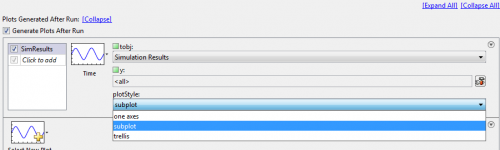Three items need to be configured for this plot type:

• tobj (which we will learn about later)
• y (used to specify which states to plot)
• and plotStyle, either one axes, subplotor trellis

The subplotoption often confuses people – and I was one of them when I started with SimBiology. Many (including myself) intuitively expect this option to allow plotting of each state in its own plot axes, i.e., in its own subplot. However, this option is used to plot the results of several simulations (from a scan or ensemble simulation) into individual subplots. How could we plot each state in a separate subplot?
A possible solution is to add a user-defined plot to the Plot Type Library. This library can be accessed from the address bar: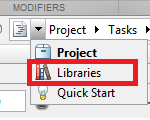In the Plot Types Library window, a list of the available Plot Types and the associated code can be found. The easiest to get started is to copy-paste one of the existing Plot Types and modify the copied code. However, here I will start with a blank new Plot Type. Let’s add a new Plot Type called State Subplot: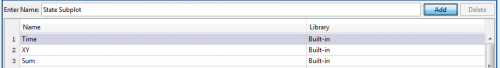Clicking on Add will automatically create a template that we can extend to create our own Plot Type:

function State_Subplot(tobj)


Let’s add a second argument containing the names of the states that we want to plot:

function State_Subplot(tobj, y)


Below the editor is a box where the arguments that should be passed into this plotting function need to be defined. The second argument y has already been added to this table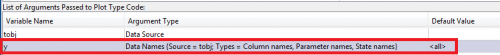These are the parameters that will later on need to be specified in the task window. There’s a choice of six argument types, the most important one for our purposes are Data Source, Data Names and Enumerations. Data Source contains the results of the simulation in the form of a SimData object or a Dataset array in the case of external data (after these have been imported into the Data Tab). tobj is already predefined as a Data Source; we will choose Data Names as the Argument Type for y with the default value set to <all>. Enumerations can be used to provide a drop-down list of string alternatives. As an example for their use, the Time plot type uses enumerations to provide the PlotStyle dialog to distinguish between one axes, subplot and trellis.

Now everything is set up and the remaining task is to code the subplot itself.
First, we will extract the simulation data from the SimData object tobj

% Get Data to be plotted
if strcmpi(y, '<all>')
[time, data, names] = getdata(tobj);
else
[time, data, names] = selectbyname(tobj, y);
end


We need to distinguish between the default option and a list of state names. selectbyname() can be used to extract simulation data for a limited number of states; getdata() can be used to separate all data into double arrays and a cell array, so that they can be easily plotted using the generic plot() command.

In the next step we need to figure out the number of rows and columns for the subplot. First, we’ll calculate the square root of the number of states to be plotted

rootnplots = sqrt(numel(names));


and then – considering that monitors typically have a 4:3 or 16:9 aspect ratio – set up the number of rows and columns so that we have more columns than rows

nrows = round(rootnplots);
ncolumns = ceil(rootnplots);


Finally, the last remaining task is to create the actual plot

for(i = 1:numel(names))
subplot(nrows,ncolumns,i)
plot(time, data(:,i));
title(names(i),'Interpreter','none');
end


We step through each state using a for loop, create a new subplot axis, plot the state values against time and put the name of the state as a title, switching off LaTeX interpretation of the title string so that underscores, for example, are not interpreted in the LaTeX way as a subscript. Axis labels and other things could also be set here. Done.

Last, you might want to share your newly created plot type with one of your colleagues. For this, click on the action button left of the address bar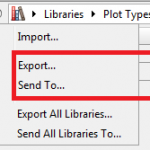and either export the plot type or send it as an email attachment directly from SimBiology. Your colleague can import the new plot type by importing the .plottype file from the same dialog. After this import, the new plot type is available from the plot list in the task window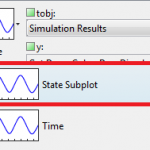Any comments/questions? Send me an email.